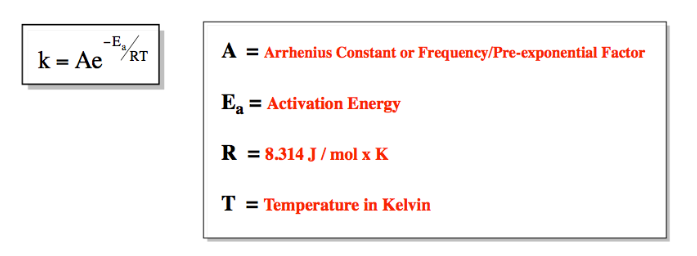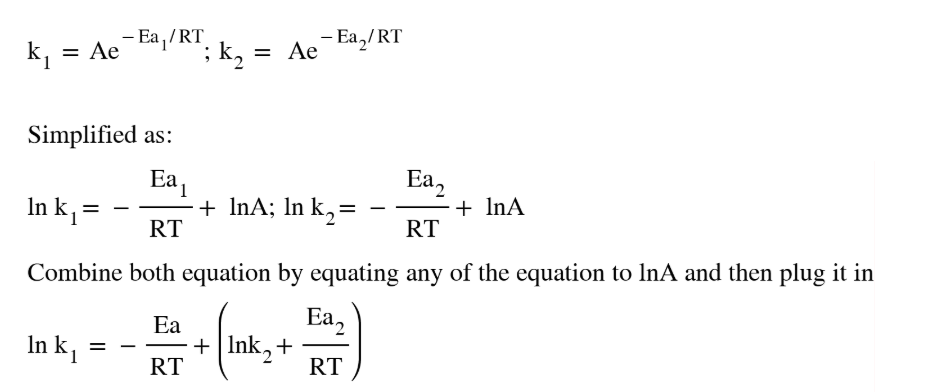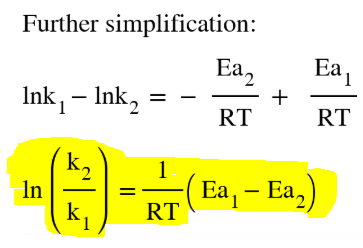# Problem: The activation energy for a reaction is changed from 184 kJ/mol to 59.0 kJ/mol at 600. K by the introduction of a catalyst. If the uncatalyzed reaction takes about 2400 years to occur, about how long will the catalyzed reaction take? Assume the frequency factor A is constant, and assume the initial concentrations are the same.

###### FREE Expert Solution
• Arrhenius Equation shows the dependence of the rate constant on the pre-exponential factor, absolute temperature and activation energy of the reaction where:• The equation for the catalyzed and uncatalyzed reaction will appear as:###### Problem Details

The activation energy for a reaction is changed from 184 kJ/mol to 59.0 kJ/mol at 600. K by the introduction of a catalyst. If the uncatalyzed reaction takes about 2400 years to occur, about how long will the catalyzed reaction take? Assume the frequency factor A is constant, and assume the initial concentrations are the same.